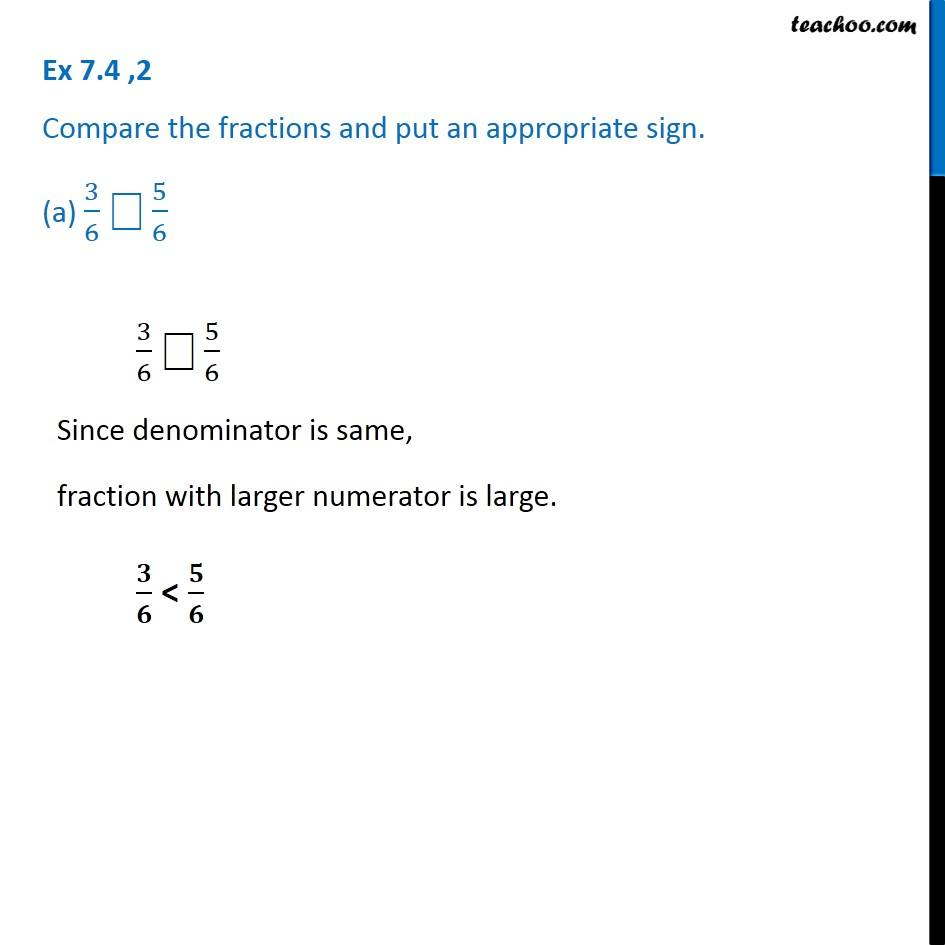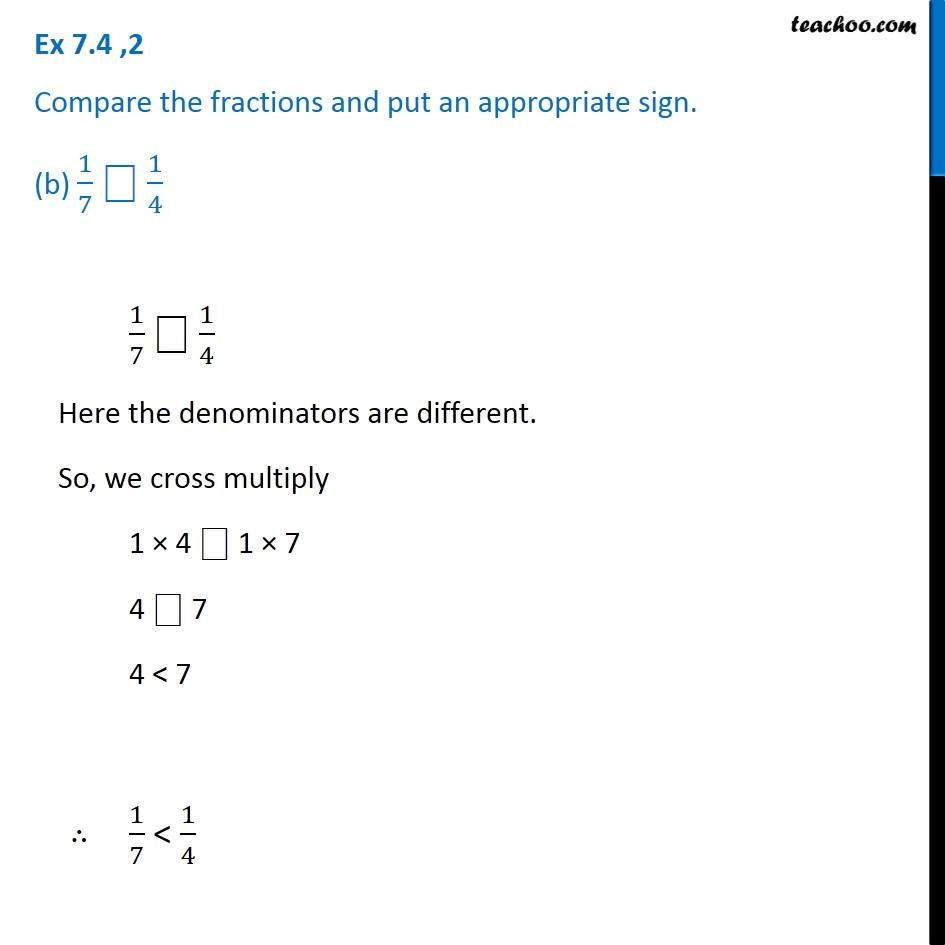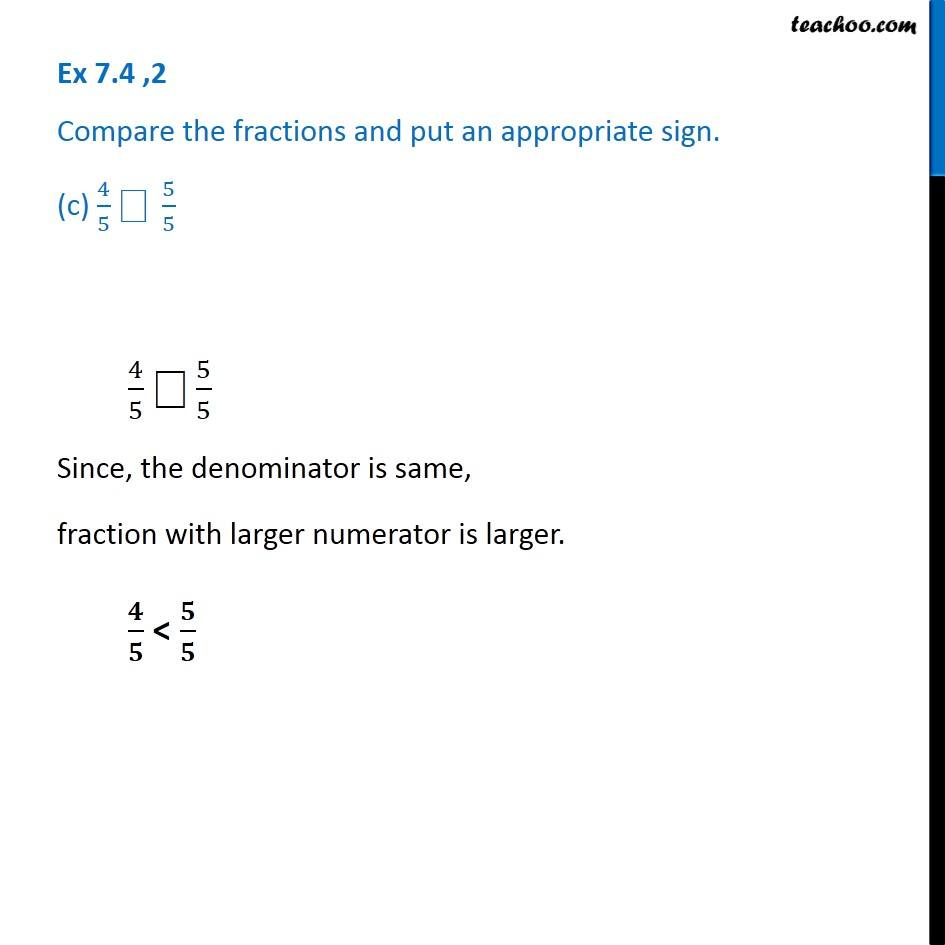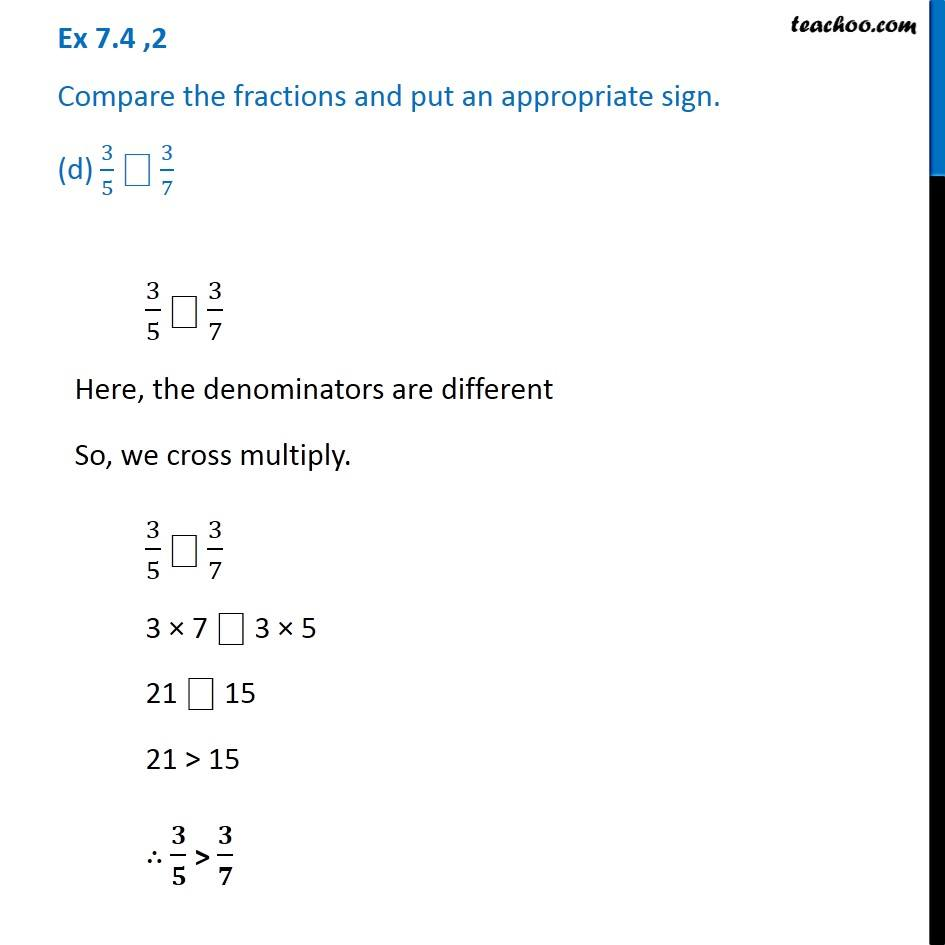Comparing fractions

Chapter 7 Class 6 Fractions
Concept wiseLearn in your speed, with individual attention - Teachoo Maths 1-on-1 Class

### Transcript

Ex 7.4 ,2 Compare the fractions and put an appropriate sign. (a) 3/6 "⎕" 5/6 3/6 "⎕" 5/6 Since denominator is same, fraction with larger numerator is large. 𝟑/𝟔 < 𝟓/𝟔 Ex 7.4 ,2 Compare the fractions and put an appropriate sign. (b) 1/7 "⎕" 1/4 1/7 "⎕" 1/4 Here the denominators are different. So, we cross multiply 1 × 4 "⎕" 1 × 7 4 "⎕" 7 4 < 7 ∴ 1/7 < 1/4 1/7 "⎕" 1/4 Here the denominators are different. So, we cross multiply 1 × 4 "⎕" 1 × 7 4 "⎕" 7 4 < 7 ∴ 1/7 < 1/4 4/5 "⎕" 5/5 Since, the denominator is same, fraction with larger numerator is larger. 𝟒/𝟓 < 𝟓/𝟓 Ex 7.4 ,2 Compare the fractions and put an appropriate sign. (d) 3/5 "⎕" 3/7 3/5 "⎕" 3/7 Here, the denominators are different So, we cross multiply. 3/5 "⎕" 3/7 3 × 7 ⎕ 3 × 5 21 ⎕ 15 21 > 15 ∴ 𝟑/𝟓 > 𝟑/𝟕